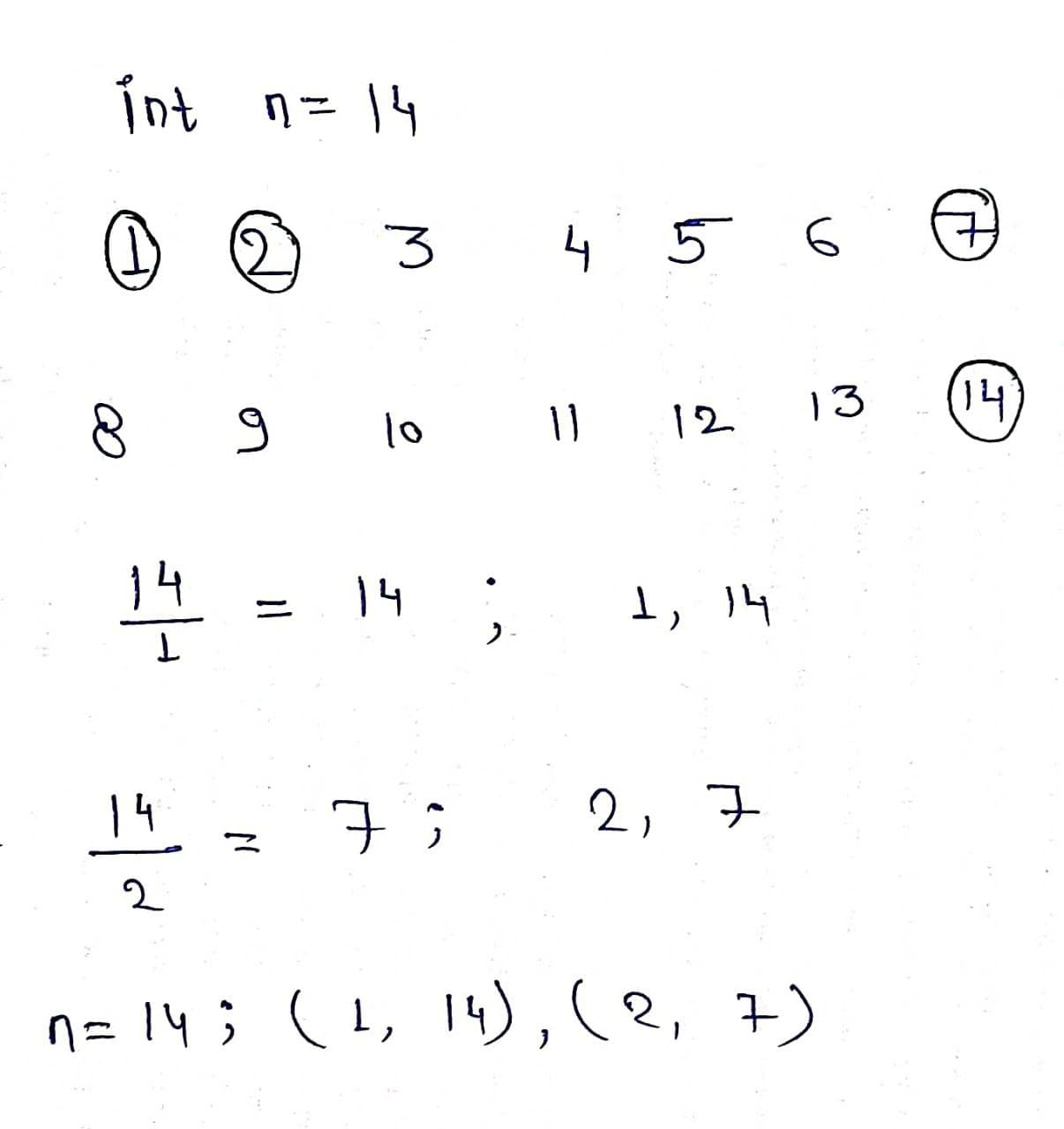# Factors of a Given Number

Problem Statement: Find all factors of a number or find all distinct divisors of a natural number.

Examples:

```Example 1:
Input: n = 6
Output: 1,2,3,6
Explanation: 6 is divisible by 1,2,3,6

Example 2:
Input: n = 9
Output: 1,3,9
Explanation: 9 is divisible by 1,3,9
```

### Solution

Disclaimer: Don’t jump directly to the solution, try it out yourself first.

Solution1: Brute force

Intuition:  We can simply use a for loop from 1 to n and check for every element that n is divisible by it or not, if it is then we will print it otherwise just skip it.

Approach:

• Use a for loop from 1 to n.
• If n is divisible by any element of the loop then print it.
• Otherwise skip it.

Code:

## C++ Code

``````#include <iostream>
using namespace std;

void Divisor(int n)
{
for (int i = 1; i <= n; i++)
if (n % i == 0)
cout <<" " << i;
}

int main()
{   int n = 6;
cout <<"Factors of "<<n<<" are: ";
Divisor(n);
return 0;
}
``````

Output: Factors of 6 are: 1 2 3 6

Time Complexity: O(N)

Space Complexity: O(1)

## Java Code

``````import java.util.*;
public class tuf {
static void Divisor(int n)
{
for (int i=1;i<=n;i++)
if (n%i==0)
System.out.print(i+" ");
}
public static void main(String args[])
{   int n = 6;
System.out.print("Factors of " + n + " are: ");
Divisor(n);
}
}
``````

Output: Factors of 6 are: 1 2 3 6

Time Complexity: O(N)

Space Complexity: O(1)

Solution2: Optimised approach

Intuition: When we thoroughly see the factors of a natural number, they always lie in pairs. For if ‘n’ is divisible by any number ‘i’ then it will also be divisible by its quotient of n/i.Approach:

• Run a for loop from 1 to SquareRoot of n.
• If n is divisible by any number i then the quotient of n/i is also divisible by n, print both i and n/i.
• Take care of the edge case when n/i=i, print only i one time, to remove duplicates.

Code:

## C++ Code

``````#include <bits/stdc++.h>
using namespace std;

void Divisor(int n)
{
for (int i=1; i<=sqrt(n); i++)
{
if (n%i == 0)
{
// If divisors are equal, print only one
if (n/i == i)
cout <<" "<< i;

else // Otherwise print both
cout << " "<< i << " " << n/i;
}
}
}
int main()
{   int n = 14;
cout <<"Factors of "<<n<<" are: ";
Divisor(n);
return 0;
}
``````

Output: Factors of 14 are: 1 14 2 7

Time Complexity: O(sqrt(N))

Space Complexity: O(1)

## Java Code

``````import java.util.*;

public class tuf {
static void Divisor(int n)
{
for (int i=1;i<=Math.sqrt(n); i++)
{
if (n%i==0)
{
// If divisors are equal, print only one
if (n/i == i)
System.out.print(" "+ i);

else // Otherwise print both
System.out.print(i+" " + n/i + " " );
}
}
}
public static void main(String args[])
{   int n = 14;
System.out.print("Factors of " + n + " are: ");
Divisor(n);;
}
}
``````

Output: Factors of 14 are: 1 14 2 7

Time Complexity: O(sqrt(N))

Space Complexity: O(1)

Special thanks to Prashant Sahu for contributing to this article on takeUforward. If you also wish to share your knowledge with the takeUforward fam, please check out this article